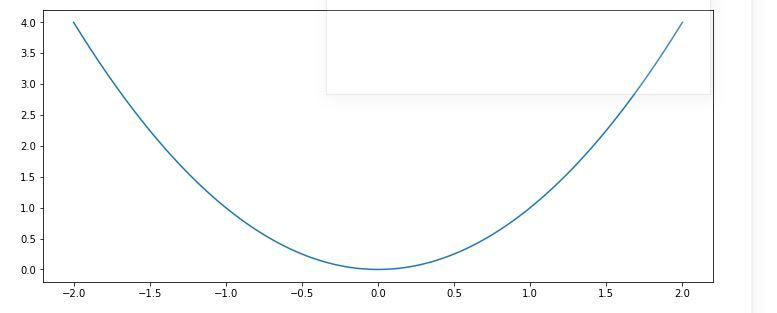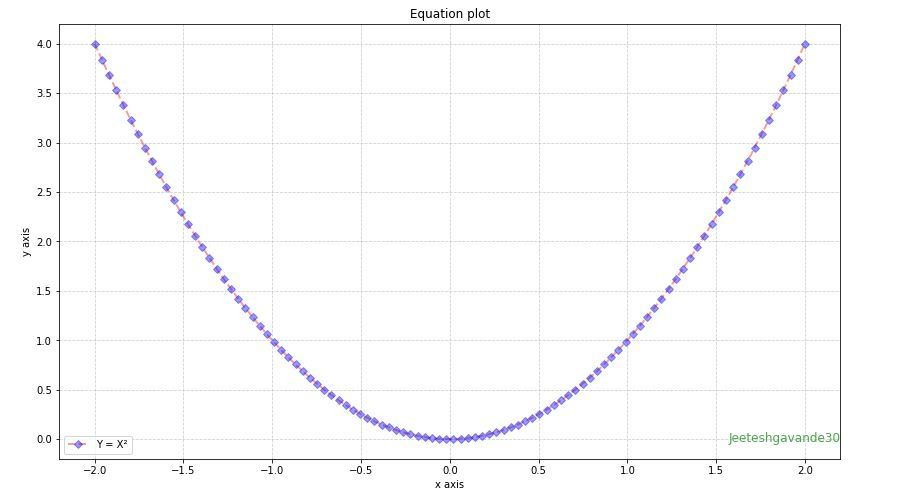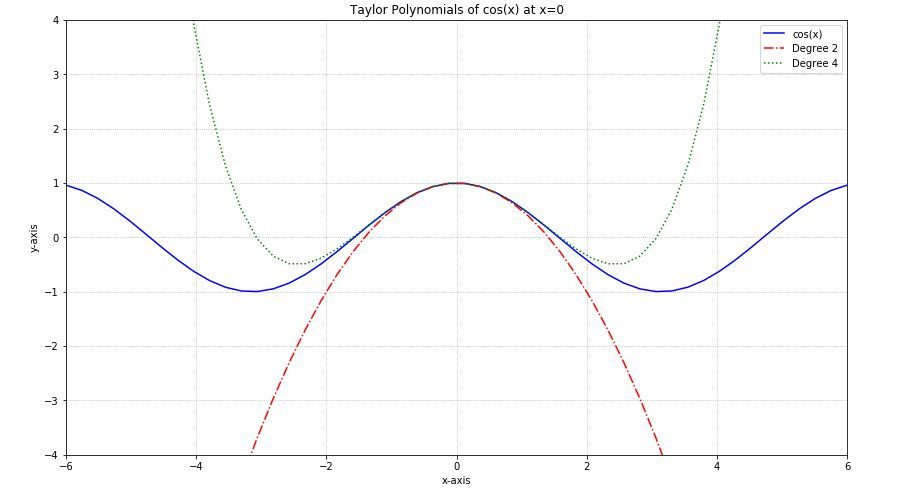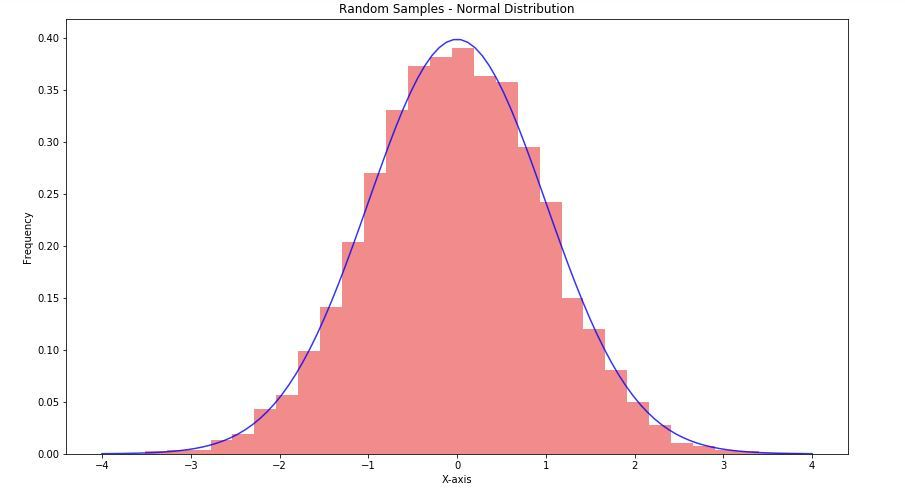# Plot Mathematical Expressions in Python using Matplotlib

For plotting equations we will use two modules Matplotlib.pyplot and Numpy. This module helps you to organize your Python code logically.

### Numpy

Numpy is a core library used in Python for scientific computing. This Python library supports you for a large, multidimensional array object, various derived objects like matrices and masked arrays, and assortment routines that makes array operations faster, which includes mathematical, logical, basic linear algebra, basic statistical operations, shape manipulation, input/output, sorting, selecting, discrete Fourier transforms, random simulation and many more operations.

### Matplotlib.pyplot

Matplotlib is a plotting library of Python which is a collection of command style functions that makes it work like MATLAB. It provides an object-oriented API for embedding plots into applications using general-purpose GUI toolkits. Each #pyplot# function creates some changes to the figures i.e. creates a figure, creating a plot area in the figure, plotting some lines in the plot area, decoration of the plot with some labels, etc.

## Plotting the equation

Lets start our work with one of the most simplest and common equation Y = X². We want to plot 100 points on X-axis. In this case, the each and every value of Y is square of X value of the same index.

 # Import libraries  import matplotlib.pyplot as plt  import numpy as np     # Creating vectors X and Y  x = np.linspace(-2, 2, 100)  y = x ** 2    fig = plt.figure(figsize = (10, 5))  # Create the plot  plt.plot(x, y)     # Show the plot  plt.show()

Output-Here note that the number of points we are using in line plot(100 in this case) is totally arbitrary but the goal here is to show a smooth graph for a smooth curve and that’s why we have to pick enough numbers depending on on the function. But be careful that do not generate too many points as a large number of points will require a long time for plotting.

### Customization of plots

There are many pyplot functions are available for the customization of the plots, and may line styles and marker styles for the beautification of the plot.Following are some of them:

Function Description
plt.xlim() sets the limits for X-axis
plt.ylim() sets the limits for Y-axis
plt.grid() adds grid lines in the plot
plt.xlabel() adds label to the horizontal axis
plt.ylabel() adds label to the vertical axis
plt.axis() sets axis properties (equal, off, scaled, etc.)
plt.xticks() sets tick locations on the horizontal axis
plt.yticks() sets tick locations on the vertical axis
plt.legend() used to display legends for several lines in the same figure
plt.savefig() saves figure (as .png, .pdf, etc.) to working directory
plt.figure() used to set new figure properties

Line Styles

Character Line style
solid line style
dashed line style
-. dash-dot line style
: dotted line style

Markers

Character Marker
. point
o circle
v triangle down
^ triangle up
s square
p pentagon
* star
+ plus
x cross
D diamond

Below is a plot created using some of this modifications:

 # Import libraries  import matplotlib.pyplot as plt  import numpy as np     # Createing vectors X and Y  x = np.linspace(-2, 2, 100)  y = x ** 2    fig = plt.figure(figsize = (12, 7))  # Create the plot  plt.plot(x, y, alpha = 0.4, label ='Y = X²',           color ='red', linestyle ='dashed',           linewidth = 2, marker ='D',            markersize = 5, markerfacecolor ='blue',           markeredgecolor ='blue')     # Add a title  plt.title('Equation plot')     # Add X and y Label  plt.xlabel('x axis')  plt.ylabel('y axis')     # Add Text watermark  fig.text(0.9, 0.15, 'Jeeteshgavande30',            fontsize = 12, color ='green',           ha ='right', va ='bottom',            alpha = 0.7)     # Add a grid  plt.grid(alpha =.6, linestyle ='--')     # Add a Legend  plt.legend()     # Show the plot  plt.show()

Output-Example 1-
Plotting a graph of the function y = Cos(x) with its Taylor polynomials of degree 2 and 4.

 # Import libraries  import matplotlib.pyplot as plt  import numpy as np     x = np.linspace(-6, 6, 50)     fig = plt.figure(figsize = (14, 8))     # Plot y = cos(x)  y = np.cos(x)  plt.plot(x, y, 'b', label ='cos(x)')     # Plot degree 2 Taylor polynomial  y2 = 1 - x**2 / 2 plt.plot(x, y2, 'r-.', label ='Degree 2')     # Plot degree 4 Taylor polynomial  y4 = 1 - x**2 / 2 + x**4 / 24 plt.plot(x, y4, 'g:', label ='Degree 4')     # Add features to our figure  plt.legend()  plt.grid(True, linestyle =':')  plt.xlim([-6, 6])  plt.ylim([-4, 4])     plt.title('Taylor Polynomials of cos(x) at x = 0')  plt.xlabel('x-axis')  plt.ylabel('y-axis')     # Show plot  plt.show()

Output-Example 2-
Generating an array of 10000 random entries sampled from normal distribution and creating a histogram along with a normal distribution of the equation:# Import libraries  import matplotlib.pyplot as plt  import numpy as np     fig = plt.figure(figsize = (14, 8))     # Creating histogram  samples = np.random.randn(10000)  plt.hist(samples, bins = 30, density = True,           alpha = 0.5, color =(0.9, 0.1, 0.1))     # Add a title  plt.title('Random Samples - Normal Distribution')     # Add X and y Label  plt.ylabel('X-axis')  plt.ylabel('Frequency')     # Creating vectors X and Y  x = np.linspace(-4, 4, 100)  y = 1/(2 * np.pi)**0.5 * np.exp(-x**2 / 2)     # Creating plot  plt.plot(x, y, 'b', alpha = 0.8)     # Show plot  plt.show()

Output-My Personal Notes arrow_drop_upCheck out this Author's contributed articles.

If you like GeeksforGeeks and would like to contribute, you can also write an article using contribute.geeksforgeeks.org or mail your article to contribute@geeksforgeeks.org. See your article appearing on the GeeksforGeeks main page and help other Geeks.

Please Improve this article if you find anything incorrect by clicking on the "Improve Article" button below.

Article Tags :

Be the First to upvote.

Please write to us at contribute@geeksforgeeks.org to report any issue with the above content.